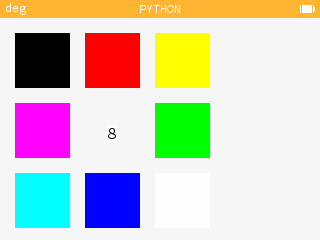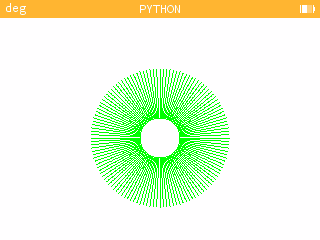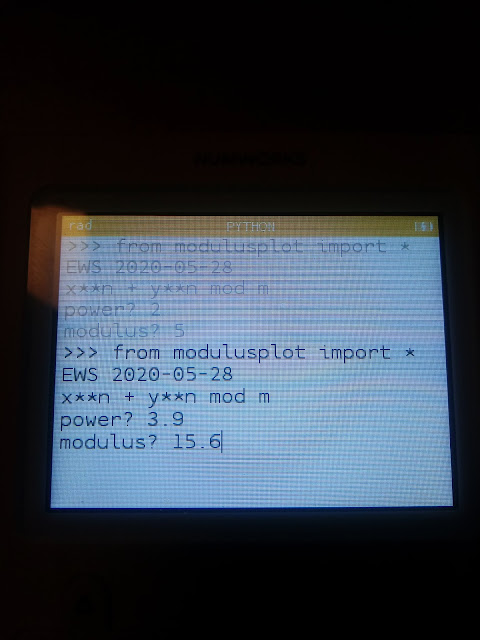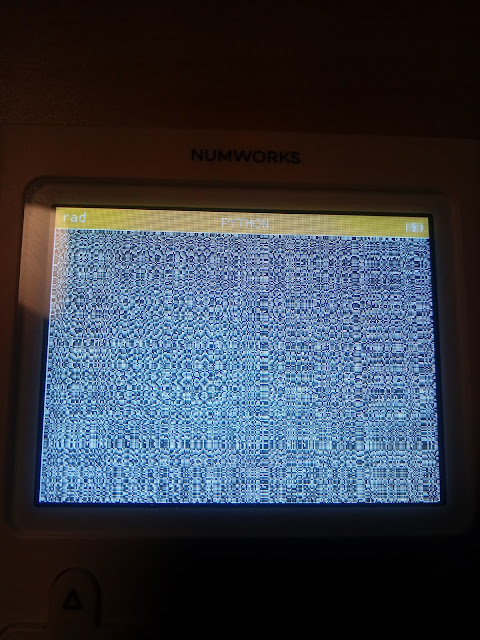## Sunday, June 28, 2020

### Numworks Python Scripts: Basic Graphics

Numworks Python Scripts:  Basic Graphics

Script:  atari.py

Draws the basic-eight color palette of the classic 1977 Atari 2600.Atari Palette Python Script

from math import *
from kandinsky import *
# 2020-05-28 atari 2600 colors
# kandinsky module

fill_rect(0,0,320,240,color(245,245,245))

fill_rect(15,15,55,55,color(0,0,0))
fill_rect(85,15,55,55,color(255,0,0))
fill_rect(155,15,55,55,color(255,255,0))
fill_rect(15,85,55,55,color(255,0,255))
fill_rect(155,85,55,55,color(0,255,0))
fill_rect(15,155,55,55,color(0,255,255))
fill_rect(85,155,55,55,color(0,0,255))
fill_rect(155,155,55,55,color(255,255,255))

draw_string("8",107,107)

Script:  firstdigit.py

The tenths digit from n random numbers is extracted and a bar chart is generated based on the results.  I recommend a sample size of at least 20.A Sample of 100 data points

from math import *
from random import *
from matplotlib.pyplot import *

# set up lists
x=[0,1,2,3,4,5,6,7,8,9]
y=[0,0,0,0,0,0,0,0,0,0]

# user iput
print("EWS 2020-05-29")
print("Bar Chart: First Digit")
print("Recommended at least 20")
n=int(input("n? "))

# generate list
for i in range(n):
s=int(random()*10)
y[s]=y[s]+1

# bar plot
h=int(n/2)
d=-int(h/4)
axis([-0.5,9.5,d,h])
bar(x,y)

# turn axis off
axis("off")

# labels at the bottom
# results at top
m=max(y)
for i in range(10):
text(i-0.25,d+1,str(i))
text(i-0.25,m+2,str(y[i]))

show()

Script:  colorfulrings.py

The script cycles through a set of nine colors, four times.   The Kandinsky module is used to generate the flowery circles as well as cycle through the colors.  This module works with integer pixels.Color rings script in progress

from math import *
from kandinsky import *
from time import *

# color lists
r=[255,255,255,0,0,0,51,128,255]
g=[0,102,255,128,255,0,102,128,255]
b=[0,0,0,0,0,255,255,128,255]

# angles
a=list(range(128))
for i in range(128):
a[i]=i/128*2*pi

# draw circles
for k in range(36):
n=int(fmod(k,9))
for j in range(50):
for i in range(128):
x=int(160+(20+j)*cos(a[i]))
y=int(120+(20+j)*sin(a[i]))
set_pixel(x,y,color(r[n],g[n],b[n]))
sleep(0.1)

Script:  modulusplot.py

Generate a pixel plot of the equation (x^n + y^n) mod mInput Screen  (n = 3.9, m = 15.6)Modulus Plot result, n = 3.9, m = 15.6

from math import *
from kandinsky import *
print("EWS 2020-05-28")
print("x**n + y**n mod m")
n=float(input("power? "))
m=float(input("modulus? "))

for x in range(320):
for y in range(240):
t=fmod(pow(x,n)+pow(y,n),m)
c=floor(t/m*255)
set_pixel(x+1,y+1,color(c,c,c))

Eddie

BLOG UPDATE:
The HP Prime:  Conversion to Binary and IEEE-754 Binary blog entry that was posted on June 27, 2020 may contain errors.  In this case, I have taken that entry back to draft status and my intention is to repost the entry as soon as I can.  Apologies for any inconvenience.

All original content copyright, © 2011-2020.  Edward Shore.   Unauthorized use and/or unauthorized distribution for commercial purposes without express and written permission from the author is strictly prohibited.  This blog entry may be distributed for noncommercial purposes, provided that full credit is given to the author.

## Sunday, June 21, 2020

### TI-84 Plus CE: Bitwise Operations

TI-84 Plus CE:  Bitwise Operations

Introduction

The programs NOT, AND, OR, and XOR are presented for the TI-84 Plus CE for integer in decimal base.

NOT

For integers x > 0, the NOT function is defined as:

Σ( 2^n * ( floor(x/2^n) mod 2 + 1 ) mod 2 ) from n = 0 to floor(log(x)/log(2))

However, observing the NOT operation on scientific calculators, the not function can  be used for integers:

-(x + 1)

TI-84 Plus CE Program NOT

"2020-05-11 EWS"
Disp "NOT(X), X>0"
Prompt X
­(X+1)→N
Disp "NOT X = ",N

Examples:

NOT 192:  Returns -193

NOT -52:  Returns 51

AND

For positive integers x, y and for x ≥ y, the AND function is defined as:

Σ( 2^n * ( [ floor(x/2^n) mod 2 ] * [ floor(y/2^n) mod 2 ] ) from n = 0 to floor(log(x)/log(2))

TI-84 Plus CE Program AND

"2020-05-11 EWS"
Disp "X AND Y, X≥Y"
Prompt X,Y
0→T
For(N,0,X-fPart(X))
(X/2^N)-fPart(X/2^N)→A
2*fPart(A/2)→A
(Y/2^N)-fPart(Y/2^N)→B
2*fPart(B/2)→B
T+2^N*A*B→T
End
Disp T

Examples:

145 AND 37:  Returns 1

226 AND 125:  Returns 96

OR

For positive integers x, y and for x ≥ y, the OR function is defined as:

Σ( 2^n *
[ [ ( floor(x/2^n) mod 2) + ( floor(y/2^n) mod 2 ) + ( floor(x/2^n) mod 2 * floor(y/2^n) mod 2) ] mod 2 ] from n = 0 to floor(log(x)/log(2))

TI-84 Plus CE Program OR

"2020-05-11 EWS"
Disp "X OR Y, X≥Y"
Prompt X,Y
0→T
For(N,0,X-fPart(X))
(X/2^N)-fPart(X/2^N)→A
2*fPart(A/2)→A
(Y/2^N)-fPart(Y/2^N)→B
2*fPart(B/2)→B
T+2^N*2*fPart((A+B+A*B)/2)→T
End
Disp T

Examples:

145 OR 37:  Returns 181

226 OR 125:  Returns 255

XOR

For positive integers x, y and for x ≥ y, the XOR function is defined as:

Σ( 2^n * [ [ floor(x/2^n) + floor(y/2^n) ] mod 2 ]  from n = 0 to floor(log(x)/log(2))

TI-84 Plus CE Program XOR

"2020-05-11 EWS"
Disp "X XOR Y, X≥Y"
Prompt X,Y
0→T
For(N,0,X-fPart(X))
(X/2^N)-fPart(X/2^N)→A
2*fPart(A/2)→A
(Y/2^N)-fPart(Y/2^N)→B
2*fPart(B/2)→B
T+2^N*2*fPart((A+B)/2)→T
End
Disp T

Examples:

145 XOR 37:  Returns 180

226 XOR 125:  Returns 159

Eddie

Source:

“Bitwise Operation”  Wikipedia.  Page last edited May 10, 2020.  Accessed on May 11, 2020.

Eddie

All original content copyright, © 2011-2020.  Edward Shore.   Unauthorized use and/or unauthorized distribution for commercial purposes without express and written permission from the author is strictly prohibited.  This blog entry may be distributed for noncommercial purposes, provided that full credit is given to the author.

## Saturday, June 20, 2020

### HP 41C: Decimal Bitwise Operations

HP 41C: Decimal Bitwise Operations

The document covers the following operations:

NOT:  for integers
AND:  for positive integers
OR:  for positive integers
XOR:  for positive integers
Simple conversions between Binary and Decimal bases (integers up to 1023)

No extra or additional modules required.  Should work for the Swiss Micros DM41 and any emulators.

Eddie

All original content copyright, © 2011-2020.  Edward Shore.   Unauthorized use and/or unauthorized distribution for commercial purposes without express and written permission from the author is strictly prohibited.  This blog entry may be distributed for noncommercial purposes, provided that full credit is given to the author.

## Sunday, June 14, 2020

### TI-84 Plus CE: Complex Recurring Sequences

TI-84 Plus CE:  Complex Recurring Sequences

The program CMPXSEQ generates a matrix table for the complex recurring series:

C = (P + B * Q) / (1 + A)

where:
P and Q are complex constants
A = z_0
B = z_1
C = z_2

Restated, this recursion series becomes:

z_2 = (P + z_1* Q) / (1 + z_0)

In its current operating system (5.4.0), the TI-84 Plus CE's recurring sequence graphing mode cannot accept complex numbers.   Therefore a program is required.  Furthermore, matrices on the TI-84 Plus CE do not handle complex numbers.   It is required to break the complex numbers into parts.   For completeness I added both the rectangular and the polar parts of complex numbers:  real part, imaginary part, radius, and angle (dependent on angle mode).

TI-84 Plus CE Program CMPXSEQ  (text)

"EWS 2020-04-28"
ClrHome
a+bi
Disp "C=(P+B*Q)/(1+A)","COMPLEX","C=Z(N), B=Z(N-1), A=Z(N-2)"
Prompt P,Q
Input "Z0?",A
Input "Z1?",B
Input "NO OF STEPS?",N
{5,N+1}→dim([E])
For(I,0,N)
(P+Q*B)/(1+A)→C
I→[E](1,I+1)
real(C)→[E](2,I+1)
imag(C)→[E](3,I+1)
abs(C)→[E](4,I+1)
angle(C)→[E](5,I+1)
B→A
C→B
End
[E]^T→[E]
Disp "RESULT = [E]","[REAL,IMAG,ABS,ANGLE]"

Results are stored in matrix E.  ^T is the transpose function.

Examples

Tables generated using the LibreOffice Numbers desktop app and results verified with the TI-84 Plus CE.

Example 1:
P = 1
Q = i
z_0 = A = 2i
z_1 = B = -2

Example 2:
P = -i
Q = 1
z_0 = A = 2i
z_1 = B = -2

Example 3:
P = 1-i
Q = 1+i
z_0 = A = 2i
z_1 = B = -2

Have a great Sunday and week,

Eddie

All original content copyright, © 2011-2020.  Edward Shore.   Unauthorized use and/or unauthorized distribution for commercial purposes without express and written permission from the author is strictly prohibited.  This blog entry may be distributed for noncommercial purposes, provided that full credit is given to the author.

## Saturday, June 13, 2020

### Graph Gallery Series with GeoGebra

Graph Gallery Series with GeoGebra

The following graphs are from one to five terms of an infinite series of the form:

Σ f(n,x) from n = 0 to ∞

I use the GeoGebra online graphing tool, it is a great online, and free, graphing, mathematics, and geometry app.  Check GeoGebra out at https://www.geogebra.org

Enjoy!

Series 1:

Σ( n * x ) from n = 0 to ∞

Series 2:

Σ( x^n * e^(-n*x) ) from n = 0 to ∞

Series 3:

Σ( x^(-n) ) from n = 0 to ∞

Series 4:

Σ( n * erf((n * x) / (n + 1)) ) from n = 0 to ∞

Series 5:

Σ( n * sin x + sin( x * n ) ) from n = 0 to ∞

Series 6:

Σ( x^(-n) * cos( x * n ) ) from n = 0 to ∞One of my favorite pictures.  I used this as a wallpaper on my PC.  Eddie

Eddie

All original content copyright, © 2011-2020.  Edward Shore.   Unauthorized use and/or unauthorized distribution for commercial purposes without express and written permission from the author is strictly prohibited.  This blog entry may be distributed for noncommercial purposes, provided that full credit is given to the author.

## Sunday, June 7, 2020

### Retro Review: Radio Shack EC-4024

Quick Facts:

Model:  EC-4024
Equivalent of:  Casio fx-50F
Type:  Scientific
Years:  approximately 1987 - 1995
Display:  10 digits
Batteries:  Solar
Logic:  Algebraic (AOS)

Memory:
Registers:  7
Constants:  9
Pre-Programmed Formulas: 23
Programming Steps: 29 between 2 slots

Features:  A Little Bit of Almost Everything

The EC-4024 has the following modes:

Mode 0:  Compute  (Main mode)
Mode 1:  Base Mode  (can be used in programs)
Mode 2:  Linear Regression (y = A + Bx) (can be used in programs)
Mode 3:  Single Deviation (one variable stats) (can be used in programs)
Mode 4:  Degrees Angle Mode

Modes 7, 8, and 9:  Fixed, Scientific, and Normal (standard) display modes, respectively.

Mode . (decimal point) sets the EC-4024 in run mode while Mode EXP sets the EC-4024 in program writing mode.

The calculator handles fractions and converts results between fractions and decimal equivalents.

The base mode has the traditional four bases (binary, octal, decimal, hexadecimal integers) with Boolean algebra (OR, AND, NOT, XOR, NXOR, NEG).

Equivalents

The Radio Shack EC-4024 is the equivalent of the Casio fx-50F calculator from the late 1980s.   There is a calculator similar to them both, which is a Casio fx-10F, however the fx-10F has an angled body and lacks the scientific constants.

Constants

Outside of the statistics mode, the shift keys of the 1 through 9 keys holds the following constants that can be recalled (1978 Japan Industrial Standard, JIS Z-8202-1978) :

1:  C: Speed of Light:  299792458 m/s
2:  h:  Plank Constant: 6.626176 * 10^-34 J s
3:  G:  Universal Gravitational Constant: 6.672 * 10^-11 N m^2 kg^2
4:  e:  Elementary Charge:  1.6021892 * 10^-19 C
5:  me:  Electron Mass: 9.109534 * 10^-34 kg
6:  u:  Atomic Mass Unit:  1.66055655 * 10^-27 kg
7:  Na:  Avogadro Constant: 6.022045*10^23 / mol
8:  k:  Boltzmann Constant: 1.380662 * 10^-23 J/K
9:  Vm:  Molar Value of Ideal Gas at Standard Temperature and Pressure: 0.02241383 m^3/mol

What is neat about this is that the units are listed next the constant name.  Example:  C(ms^-1) is the shift function of the 1 key.

Statistics Variables

The following are the variables used in the statistics mode.  The functions for the y values, A, B, and r are available to LR mode only.  The left parenthesis key becomes the comma key, and the right parenthesis key is the predict key (LR mode only).

Shift 1:  average of x values
Shift 2:  population deviation of x values
Shift 3:  sample deviation of x values
Shift 4:  averages of y values
Shift 5:  population deviation of y values
Shift 6:  sample deviation of y values
Shift 7:  A:  intercept
Shift 8:  B:  slope
Shift 9:  r:   correlation
Kout 1:  Σx^2
Kout 2:  Σx
Kout 3:  n (number of data points)
Kout 4:  Σy^2
Kout 5:  Σy
Kout 6:  Σxy

Formulas

There are 23 formulas.  The character that is on the far left side of the screen prompts for the variable to be entered.   For a full list of formulas, please see the link below to download the manual.  The fx-10F manual also applies to both fx-50F and EC-4024 as well.

Enter required values with the [RUN] key, not the [ = ] key.

Programming

The program capacity is only 29 steps.  Thankfully, most of the shift/Kout/Mode key combinations are merged keystrokes.  For example, [MODE] [ 5 ] takes only one step, and so does [SHIFT] [ 1/x ] (for x!).  However, each press of a number key in terms of entering numbers takes a step, so to enter a numerical constant for example, 400, takes three steps ([ 4 ], [ 0 ], [ 0 ]).  With only 29 steps, economy and storing constants prior to running programs is key.

There are a few programming tools:

x>0:  Tests whether the value in the display is positive.  If so, the program loops back to the first step in the program.

x≤M:  Tests whether the value in the display is less than or equal to the value in the M register.  If the test is true, the program loops back to the first step in the program.

ENT (the [RUN] key in write mode):  Prompts the user to enter a value.  You'll need to have a valid number entered to continue programming.

HLT (the [SHIFT] [RUN] key in write mode): Stops the calculator to show the display mid-program.  Press the [RUN] key to continue.

ALPHA (the [FMLA] key in write mode):  Prompts the user to enter a value with a prompt "A?" through "F?".   The values are stored here are stored in registers 1 through 6, respectively.

A → K1, B → K2, C → K3, D → K4, E → K5, F → K6

Sample Program:  Use the formula below to estimate gravity:

g = G * mass / radius^2 = G * A / B^2

G is the Gravitational Constant (use [SHIFT] [ 3 ])
Let A = mass

Program:  P1/P2 (you designate which program area to use)

SHIFT  3    (G)
×
FMLA  a b/c  (A?)
÷
FMLA  ° ' ''  (B?)
(enter a non-zero number here, like 1)
x^2
=

Test:  Press P1/P2

Enter 5.96E24 at the A? prompt
Enter 6.38E6 at the B? prompt

Result:  9.76924362

All programming is done blind.  The only edit function is that during programming, pressing [SHIFT] [ C ] erases the last step.

The program space can be erased by entering a number program from scratch or prior to choosing which program slot, pressing [SHIFT] [ C ].  A little confusing.

Verdict

The keyboard is very clean and organized.  Despite the lack of steps, the EC-4024 is a great calculator and programmable scientific calculators that run on solar power are far and few between.  I was lucky to find the EC-4024 because usually fx-10F, fx-50F, and EC-4024 are either not available or command a medium to high price.

Source:
Casio "fx-10F/fx-50F Scientific Calculator" manual.

Eddie

All original content copyright, © 2011-2020.  Edward Shore.   Unauthorized use and/or unauthorized distribution for commercial purposes without express and written permission from the author is strictly prohibited.  This blog entry may be distributed for noncommercial purposes, provided that full credit is given to the author.

## Saturday, June 6, 2020

### HP 42S/DM42/Free42: X-Ray Spectrometric Calculations

HP 42S/DM42/Free42:   X-Ray Spectrometric Calculations

Introduction

The program XRAY contains a menu of several calculations involving flat crystal spectronomers, which include:

[ >EW ]:  Energy wavelength conversion, where E * λ = 12.39852.  E = energy and λ = wavelength

[ →N ]:  Store n, where n is the nth crystal.   You can calculate for any crystal and have any number of crystals for this program.

[ANGLE]:  Calculates the double diffraction angle, in degrees, of any crystal given n, E, and λ by using the Bragg Equation:

θ = 2 * asin( (12.39852 * n) / (E * d) )

where d = the spacing of the crystal planes

You will be prompted for E and d each time.

[E KEV]:  Uses the Bragg Equation to calculate energy (E), given n, d, and θ.  You will be prompted for d and θ each time.

[ENRGY]:  Calculates the energy resolving power (δ) at an X-ray given its line energy (E), double refraction angle (θ), and angular divergence of the collimator (δ).

ENERGY  = (E * 1000 * δ° * π / 180) / tan θ

Enter δ in degrees.

HP 42S/DM42/Free42 Program: XRAY

This program is based on the HP 11C/HP 15C program as published by Török (see source)

00 { 241-Byte Prgm }
01▸LBL "XRAY"
02 DEG
03▸LBL 00
04 ">EW"
05 KEY 1 GTO 01
06 "→N"
07 KEY 2 GTO 02
08 "ANGLE"
09 KEY 3 GTO 03
10 "E KEV"
11 KEY 4 GTO 04
12 "ENRGY"
13 KEY 5 GTO 05
14 "EXIT"
15 KEY 6 GTO 06
17▸LBL 07
18 STOP
19 GTO 07
20▸LBL 01
21 EXITALL
22 1/X
23 12.39852
24 ×
25 STOP
26 GTO 00
27▸LBL 02
28 EXITALL
29 STO 01
30 GTO 00
31▸LBL 03
32 EXITALL
33 12.39852
34 RCL× 01
35 "E?"
36 PROMPT
37 ÷
38 "2d?"
39 PROMPT
40 ÷
41 ASIN
42 2
43 ×
44 "DBL ANGLE="
45 AVIEW
46 STOP
47 GTO 00
48▸LBL 04
49 EXITALL
50 12.39852
51 RCL× 01
52 "2d?"
53 PROMPT
54 ÷
55 "DBL ANGLE?"
56 PROMPT
57 2
58 ÷
59 SIN
60 1/X
61 ×
62 "E="
63 AVIEW
64 STOP
65 GTO 00
66▸LBL 05
67 EXITALL
68 "DBL ANGLE?"
69 PROMPT
70 2
71 ÷
72 TAN
73 1/X
74 "DELTA?"
75 PROMPT
77 ×
78 "E?"
79 PROMPT
80 ×
81 1ᴇ3
82 ×
83 "ENERGY="
84 AVIEW
85 STOP
86 GTO 00
87▸LBL 06
89 EXITALL
90 .END.

Note N is stored in Register R01.  Every other memory register can be used.

Example

For a two-crystal spectrometer with properties:

Crystal 1 (n = 1):  6.285 Å (angstrom)  (d)
Crystal 2 (n = 2):  7.285 Å

Angular divergence:  0.31°   (δ)

An M_α  wave with wavelength 3.415 Å enters the system.  Find the energy of this M_α wave and the double refraction angle for each crystal.  Also was the energy-resolving power of each of the crystals.

[ XEQ ] (XRAY)

3.415 ( >EN )     Result:  E = 3.63061 keV  (store this in R00)
[STO] 00

1st Crystal

[ R/S ]
1 ( →N ) (ANGL)
"E?"  [RCL] 00 [R/S]
"2d?"  6.285 [R/S]

Result:  DBL ANGLE = 65.82494

(ENRGY)
"DBL ANGLE?" [ R/S ] (since θ is still on the X stack)
"DELTA?"  0.31 [STO] 02 [R/S]
"E?"  [RCL] 00 [R/S]

Result:  ENERGY= 30.34969 eV

2nd Crystal

[ R/S ]
2 ( →N ) (ANGL)
"E?"  [RCL] 00 [R/S]
"2d?"  7.285 [R/S]

Result:  DBL ANGLE = 139.28586

(ENRGY)
"DBL ANGLE?" [ R/S ] (since θ is still on the X stack)
"DELTA?"  [RCL] 02 [R/S]
"E?"  [RCL] 00 [R/S]

Result:  ENERGY= 7.28859eV

Source:

Török, I. (1990), Line identification and other simple wavelength‐dispersive x‐ray spectrometric calculations using a pocket calculator. X‐Ray Spectrom., 19: 159-161. doi:10.1002/xrs.1300190314

Eddie

All original content copyright, © 2011-2020.  Edward Shore.   Unauthorized use and/or unauthorized distribution for commercial purposes without express and written permission from the author is strictly prohibited.  This blog entry may be distributed for noncommercial purposes, provided that full credit is given to the author.

### TI-Nspire: Templates to Plot Functions, Parametric Equations, and Sequences

TI-Nspire:  Templates to Plot Functions, Parametric Equations, and Sequences Introduction The following are sample templates to plot functi...